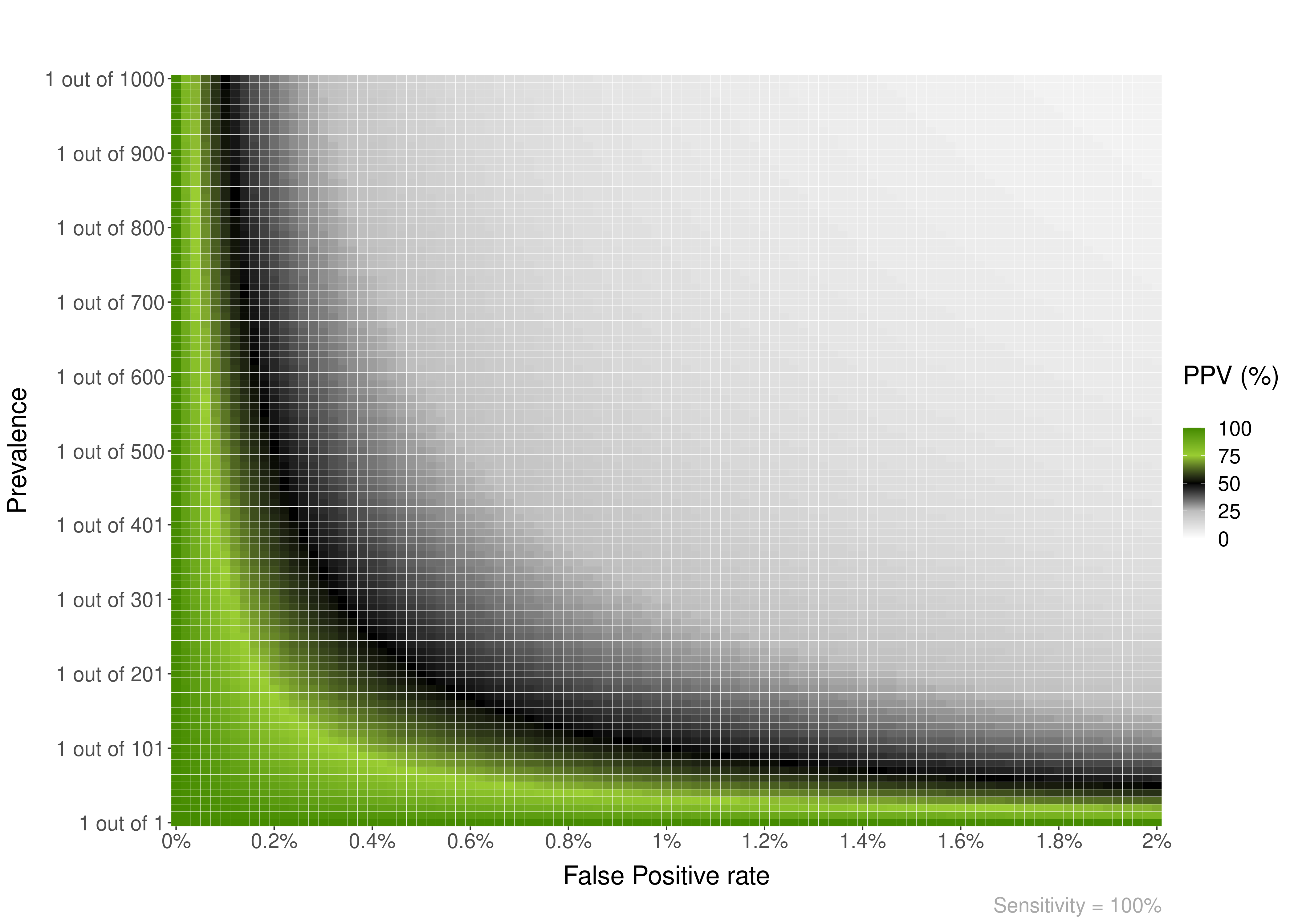# Bayesian reasoning in medical contexts

This package includes a few functions to plot and help understand Positive and Negative Predictive Values, and their relationship with Sensitivity, Specificity and Prevalence.

• The Positive Predictive Value of a medical test is the probability that a positive result will mean having the disease. Formally p(Disease|+)
• The Negative Predictive Value of a medical test is the probability that a negative result will mean not having the disease. Formally p(Healthy|-)

The BayesianReasoning package has three main functions:

• PPV_heatmap(): Plot heatmaps with PPV or NPV values for the given test and disease parameters.
• PPV_diagnostic_vs_screening(): Plots the difference between the PPV of a test in a diagnostic context (very high prevalence; or a common study sample, e.g. ~50% prevalence) versus a screening context (lower prevalence).
• min_possible_prevalence(): Calculates how high should the prevalence of a disease be to reach a desired PPV given certain test parameters.

If you want to install the package can use: `remotes::install_github("gorkang/BayesianReasoning")`. Please report any problems you find in the Issues Github page.

There is a shiny app implementation with most of the main features available.

## PPV_heatmap()

Plot heatmaps with PPV or NPV values for a given specificity and a range of Prevalences and FP or FN (1 - Sensitivity). The basic parameters are:

• Min_Prevalence: Min prevalence in y axis. “Min_Prevalence out of y”
• Max_Prevalence: Max prevalence in y axis. “1 out of Max_Prevalence”
• Sensitivity: Sensitivity of the test
• Max_FP: FP is 1 - specificity. The x axis will go from FP = 0% to Max_FP
• Language: “es” for Spanish or “en” for English
``````
PPV_heatmap(Min_Prevalence = 1,
Max_Prevalence = 1000,
Sensitivity = 100,
Max_FP = 2,
Language = "en")``````### NPV

You can also plot an NPV heatmap with PPV_NPV = “NPV”.

``````
PPV_heatmap(PPV_NPV = "NPV",
Min_Prevalence = 800,
Max_Prevalence = 1000,
Sensitivity = 80,
Max_FP = 5,
Language = "en")``````